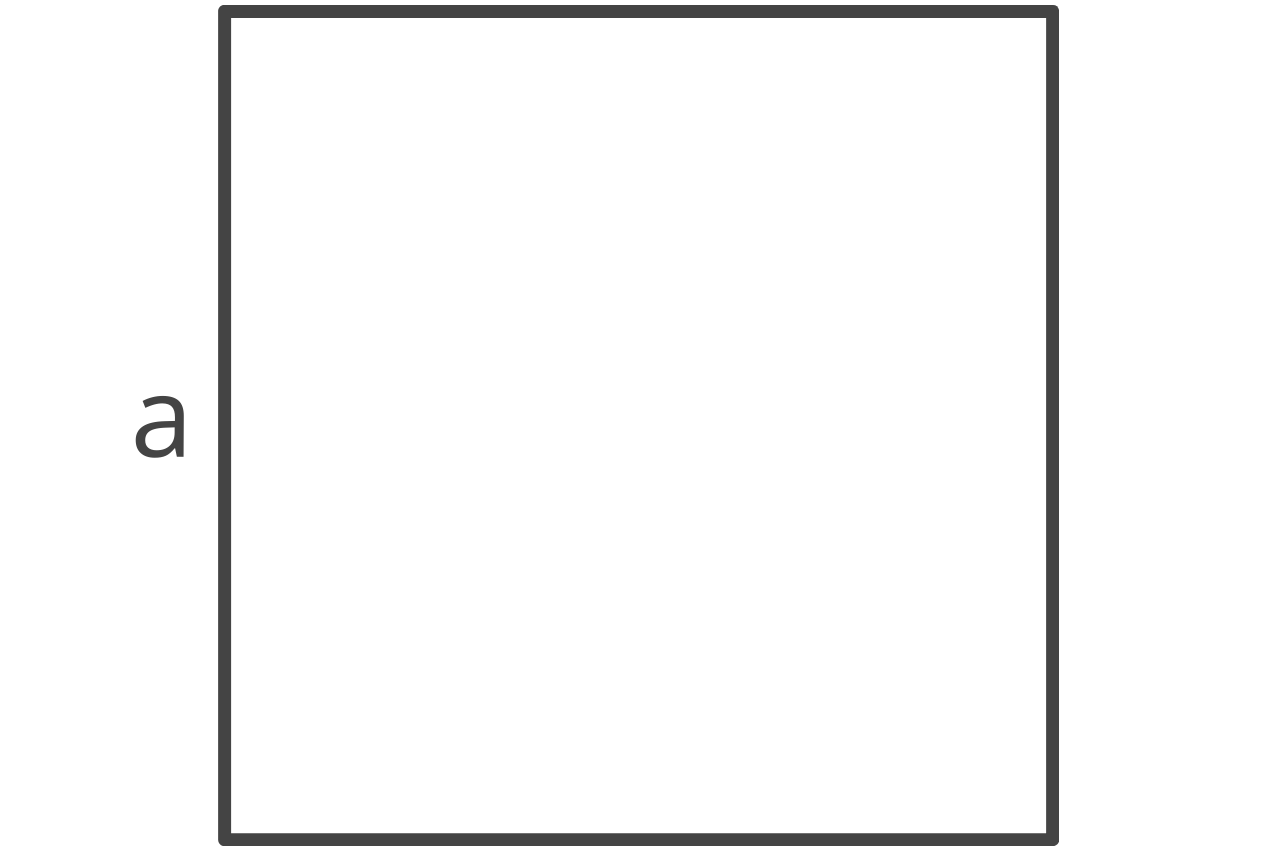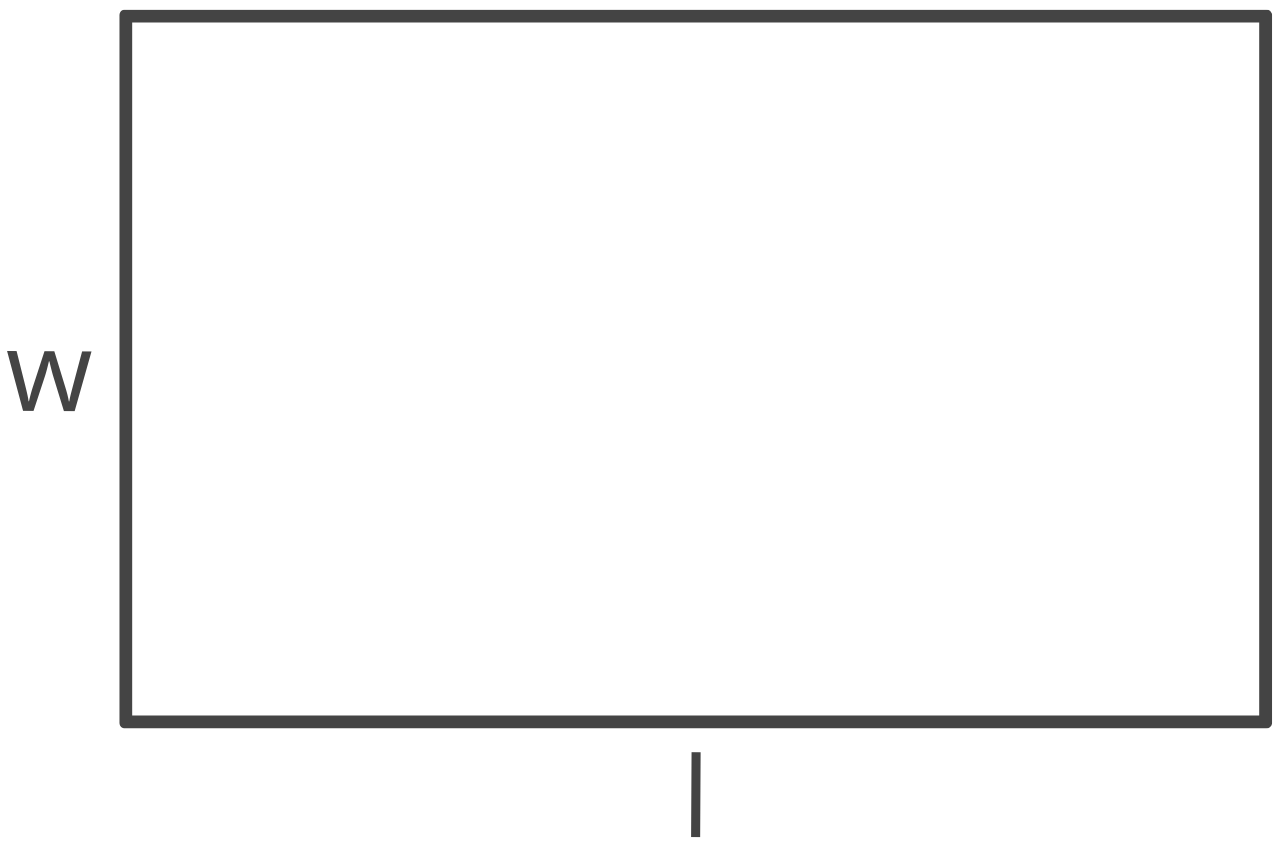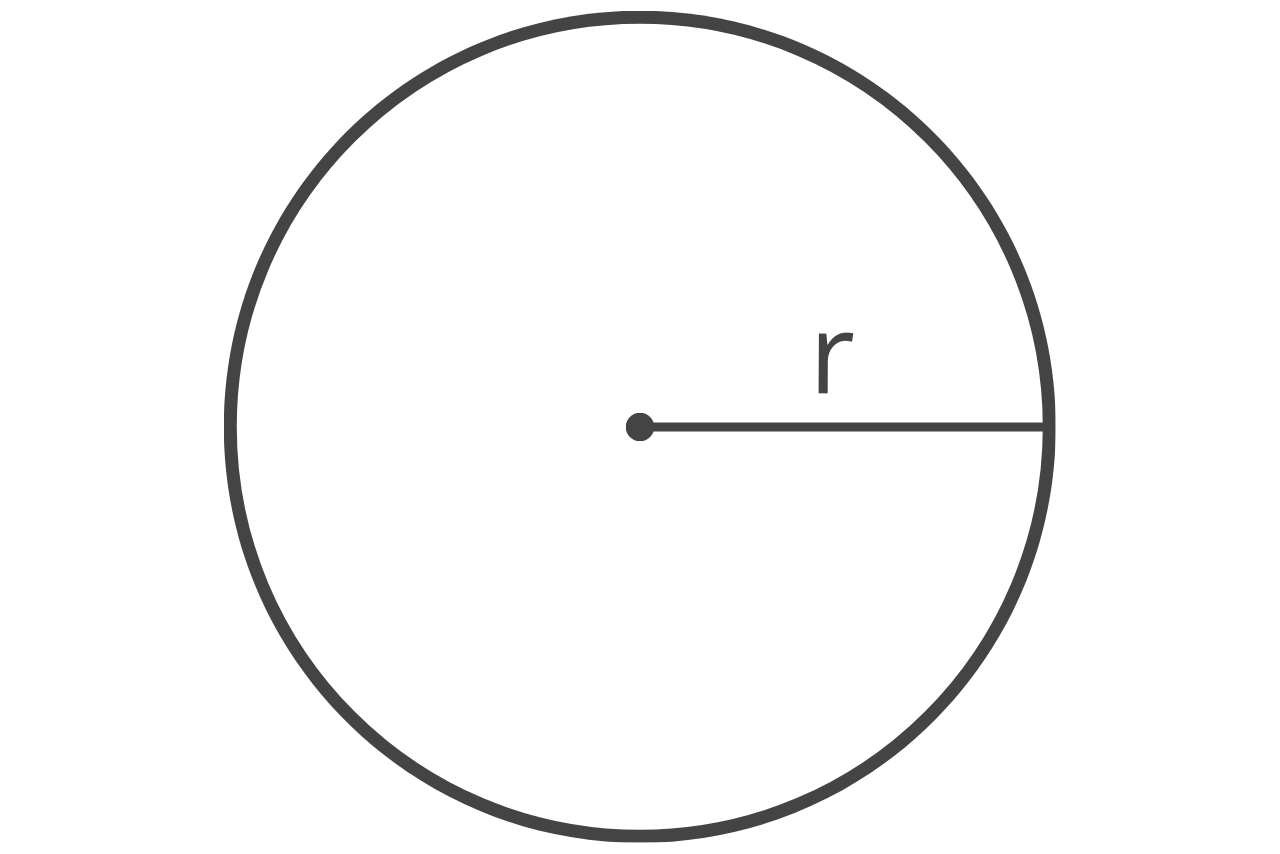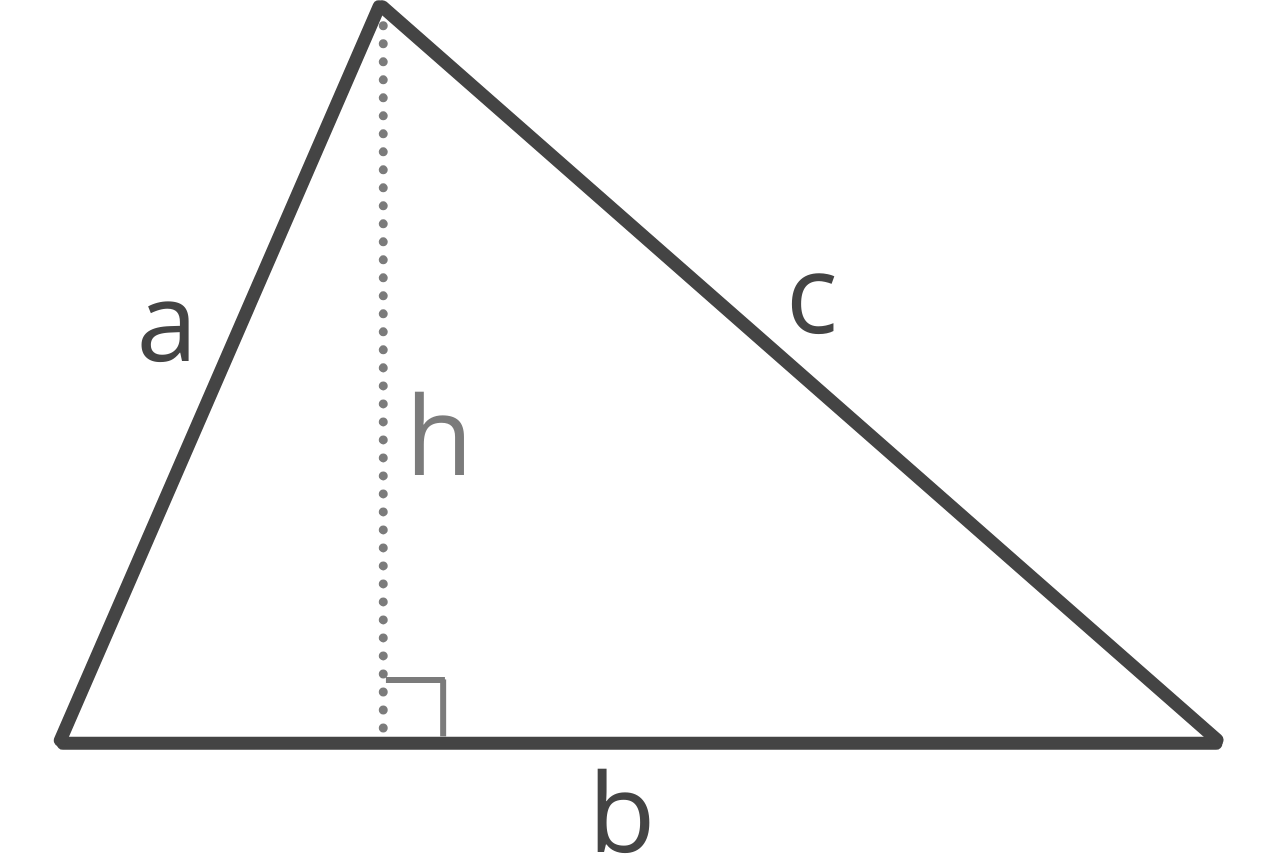# Area Conversion CalculatorsConvert one unit of area to another. Start by selecting the area unit to convert from.
US Customary
SI Units
Other Metric Units

## What is Area

Area measurements indicate the total size of a 2 dimensional shape. Put another way, area is the amount of space within the boundaries of 2 dimensional shapes, such as a rectangle or square.

When finding area, it is crucial to use the same unit of measure for all measurements. Area measurements are commonly used for construction material estimates and to identify property sizes.

## Common Area Formulas

Area is commonly found by multiplying the length of a space by the width of a space. That assumes that the space is square or rectangular. Calculating the area of a border involves subtracting the area of the inner space from the area of the outer space to find the remaining area of the border.

Finding the area of a circle or triangle requires a different formula altogether. Check out the formulas below to find out how to calculate the area of a shape. Check out our area calculator to find the area of many different shapes.### Square

area = length × width### Rectangle

area = length × width### Circle

area = π × (diameter ÷ 2)2

π = 3.14159265359### Triangle

area = (1/4) ×
(a+b+c) × (b+c-a) × (c+a-b) × (a+b-c)

## Units of Area Measurement

Units of area include square miles, acres, square yards, square feet, square inches, square kilometers, square meters, square centimeters, square millimeters, hectares, and ares.

## Common Area Measurement Conversions

Square Feet to square yards area conversion
1 square foot is equal to 0.111111 square yards
Square Yards to square feet area conversion
1 square yard is equal to 9 square feet
Square Feet to square meters area conversion
1 square foot is equal to 0.092903 square meters
Square Meters to square feet area conversion
1 square meter is equal to 10.76391 square feet
Square Kilometers to square miles area conversion
1 square kilometer is equal to 0.386102 square miles
Square Miles to square kilometers area conversion
1 square mile is equal to 2.589988 square kilometers
Square Centimeters to square inches area conversion
1 square centimeter is equal to 0.155 square inches

## Area Unit Conversion Table

Equivalent area metric and imperial measurements
Convert From Square Mile Acre Square Yard Square Foot Square Inch Square Kilometer Square Meter Square Centimeter Square Millimeter Hectare Are
1 square mile = 1 sq mi 640 ac 3,097,600 sq yd 27,878,400 sq ft 4,014,489,599 sq in 2.589988 sq km 2,589,988 sq m 25,899,881,100 sq cm 2,589,988,110,000 sq mm 258.998811 ha 25,900 a
1 acre = 0.001563 sq mi 1 ac 4,840 sq yd 43,560 sq ft 6,272,640 sq in 0.004047 sq km 4,047 sq m 40,468,564 sq cm 4,046,856,422 sq mm 0.404686 ha 40.468564 a
1 square yard = 0.00000032283 sq mi 0.000207 ac 1 sq yd 9 sq ft 1,296 sq in 0.00000083613 sq km 0.836127 sq m 8,361 sq cm 836,127 sq mm 0.000083613 ha 0.008361 a
1 square foot = 0.00000003587 sq mi 0.000022957 ac 0.111111 sq yd 1 sq ft 144 sq in 0.000000092903 sq km 0.092903 sq m 929.0304 sq cm 92,903 sq mm 0.0000092903 ha 0.000929 a
1 square inch = 0.0000000002491 sq mi 0.00000015942 ac 0.000772 sq yd 0.006944 sq ft 1 sq in 0.00000000064516 sq km 0.000645 sq m 6.4516 sq cm 645.16 sq mm 0.000000064516 ha 0.0000064516 a
1 square kilometer = 0.386102 sq mi 247.105381 ac 1,195,990 sq yd 10,763,910 sq ft 1,550,003,100 sq in 1 sq km 1,000,000 sq m 10,000,000,000 sq cm 1,000,000,000,000 sq mm 100 ha 10,000 a
1 square meter = 0.0000003861 sq mi 0.000247 ac 1.19599 sq yd 10.76391 sq ft 1,550 sq in 0.000001 sq km 1 sq m 10,000 sq cm 1,000,000 sq mm 0.0001 ha 0.01 a
1 square centimeter = 0.00000000003861 sq mi 0.000000024711 ac 0.00012 sq yd 0.001076 sq ft 0.155 sq in 0.0000000001 sq km 0.0001 sq m 1 sq cm 100 sq mm 0.00000001 ha 0.000001 a
1 square millimeter = 0.0000000000003861 sq mi 0.00000000024711 ac 0.000001196 sq yd 0.000010764 sq ft 0.00155 sq in 0.000000000001 sq km 0.000001 sq m 0.01 sq cm 1 sq mm 0.0000000001 ha 0.00000001 a
1 hectare = 0.003861 sq mi 2.471054 ac 11,960 sq yd 107,639 sq ft 15,500,031 sq in 0.01 sq km 10,000 sq m 100,000,000 sq cm 10,000,000,000 sq mm 1 ha 100 a
1 are = 0.00003861 sq mi 0.024711 ac 119.599005 sq yd 1,076 sq ft 155,000 sq in 0.0001 sq km 100 sq m 1,000,000 sq cm 100,000,000 sq mm 0.01 ha 1 a

You might also like our other measurement calculators.